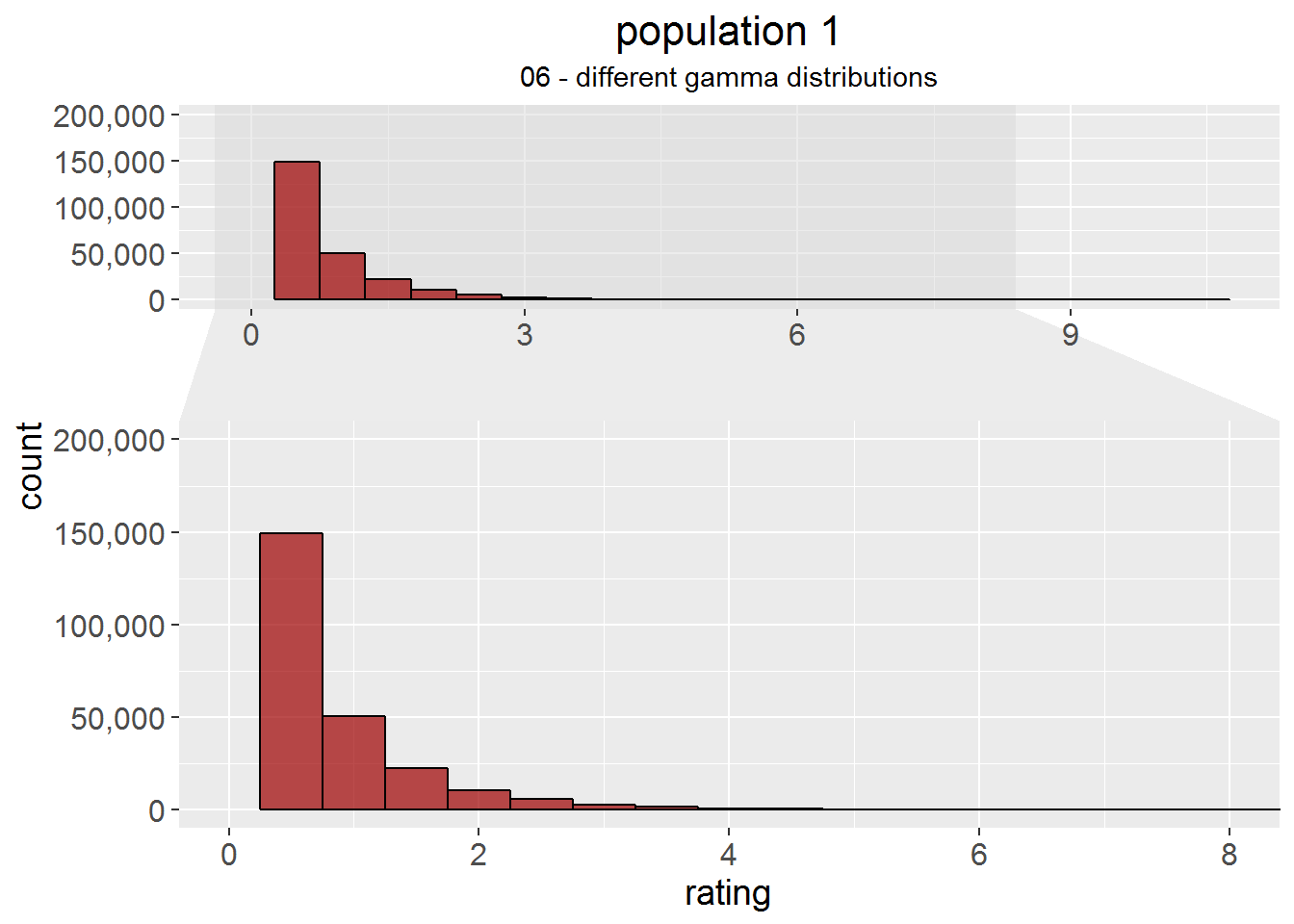## Prologue

To explain how p-values work on my German science blog, I compared the simulated ratings of two burger restaurants with each other. (Update: I did an English version of the original post. Find it here. Both restaurants were rated on a scale from 1 - 10. Then, someone on Twitter asked if it wouldn’t have been appropriate to use a nonparametric test instead of the t-test I applied, as the ratings were ordinal data. However, as I had drawn fictional data from a normal distribution, I knew it was interval data. Furthermore, I remembered the paper by Fagerland (2012) which showed the Wilcoxon test to suck for skewed data with increasing sample size - while the t-test was unaffected.

Okay, to be precise, the Wilcoxon test did not “suck”, but rather answered the different question of Prob(X < Y) = .5 instead of if the groups differ in medians. However, I don’t think many people know that (I didn’t before I read Fagerland’s paper) and are - like me - just told in their statistic lectures to calculate a Wilcoxon test when the assumptions for a t-test are not met. This can lead to … quite unintended results, as we’ll see.

For by blog however, I just wanted to prove that the t-test was the appropriate choice for my simulation (#1). At first. However, I was surprised that it didn’t fail, even if I converted the data to be plain ugly ordinal (#2). Then, things got out of hand and I turned to the holy war to bring. That. Wilcoxon. Test. Down.

Make sure to read Fagerland’s paper for a far more rigorous and systematic approach with theoretical background. Find the code for my simulations in this GitHub repo. But for now:

This is Sparta.

### The simulation

A population of 10 million ratings is generated for each restaurant. Then, samples of the following sizes (per restaurant) are drawn: 10, 50, 100, 200, 500, 1000, 2000, 3000, 4000. For each sample size, 10,000 samples of the respective size are drawn. Then, the two restaurants are compared with both, a t-test (Welch test) and a Wilcoxon rank sum (Mann Whitney U) test for independent samples. Later, I added a permutation test (`independence_test` (`distribution = "approximate"`) from the package `coin`) and a bootstrapped t-test (`boot.ttest2` from the package `Rfast`). See “Under Construction”.

In seven different scenarios, parameters of the underlying populations are varied to examine the robustness of the two tests.

## Under Construction

When Dale Barr came up with the very valid remark that the superior performance of the t-test might be due to `var.equal = FALSE`, I added another t-test with `var.equal = TRUE`. And then, quite a lot of people (e.g. Anders Eklund, David Colquhoun and Devin Didericksen) pointed out that a permutation test would have been a good call. However, there was also the objection that due to the different distributions, observations are not interchangeable and bootstrapping might work better. Hence, I added another round with a `independence_test` (`distribution = "approximate"`) from the package `coin`. And one more with `boot.ttest2` from the package `Rfast`. Lars Juhl Jensen pointed out that in some of my scenarios, the means of my populations are the same, but the medians differ. So, I’m fixing that for the next runs.

This requires the analyses to be re-run, which takes some time. I’ll update stuff step by step.

### TO DO

• Permutation test and bootstrap from scenario 5 onwards.
• Same median for populations 5 - 7
• Turns out, this is harder than it looks. I found a function meant to create something like this (and cheated a bit with rounding), but the distribution looks rather normal than lognormal. I included the example as 04a and keep working on the last scenarios.

## 01 - default

Normal distribution; integers from 1 - 10. Population 1 and 2 are identical. Both populations: \(M = 5.50\), \(SD = 2.29\), median = 6, skewness = 0.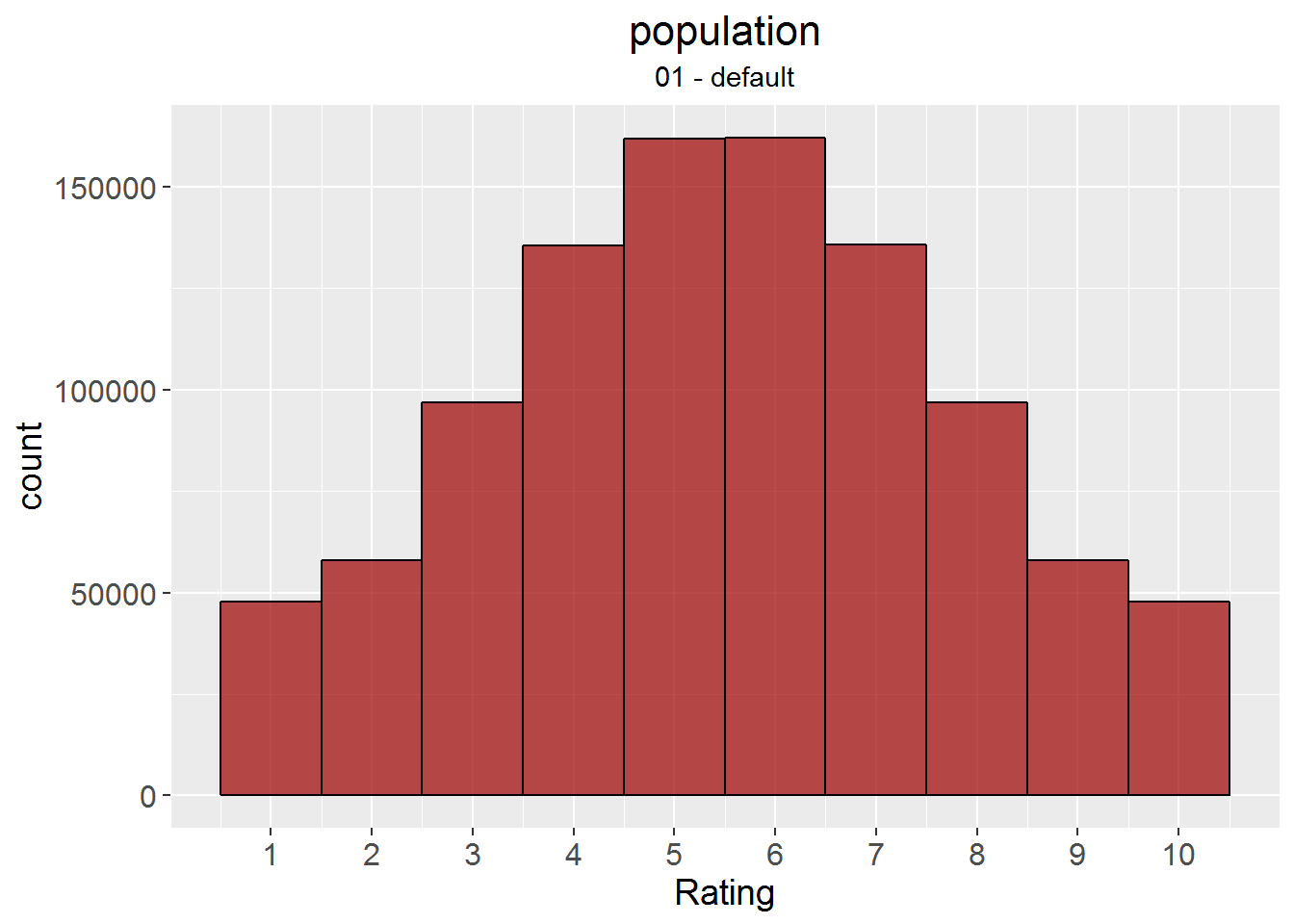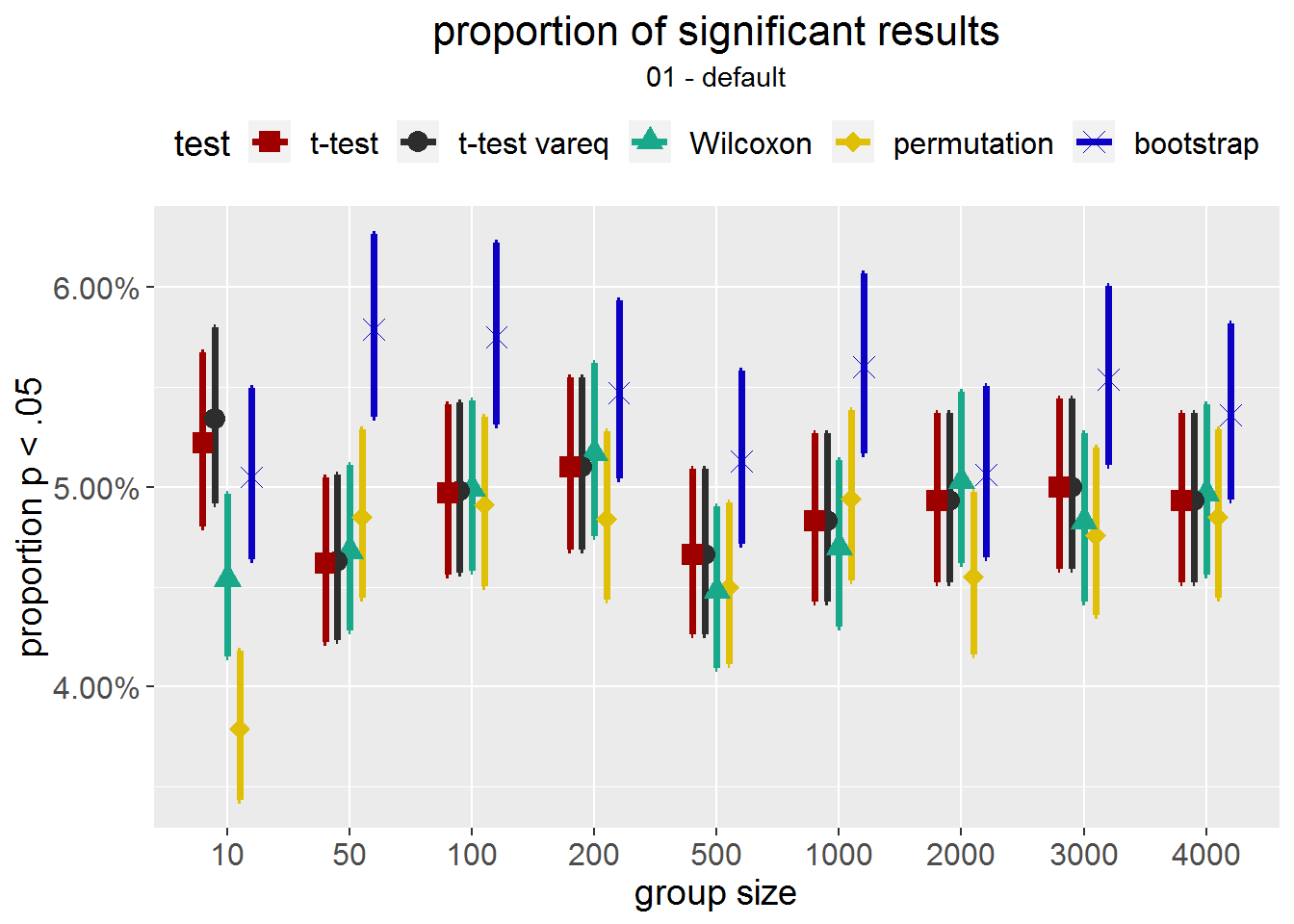## 02 - ordinalskal scale

Same populations as in #01, but converted to an ugly ordinal scale according to the following catagories:

old rating label new rating
1 - 4 poor 1
5 - 8 good 2
9 - 10 excellent 3

Both populations: \(M = 1.77\), \(SD = 0.62\), median = 2, skewness = 0.21.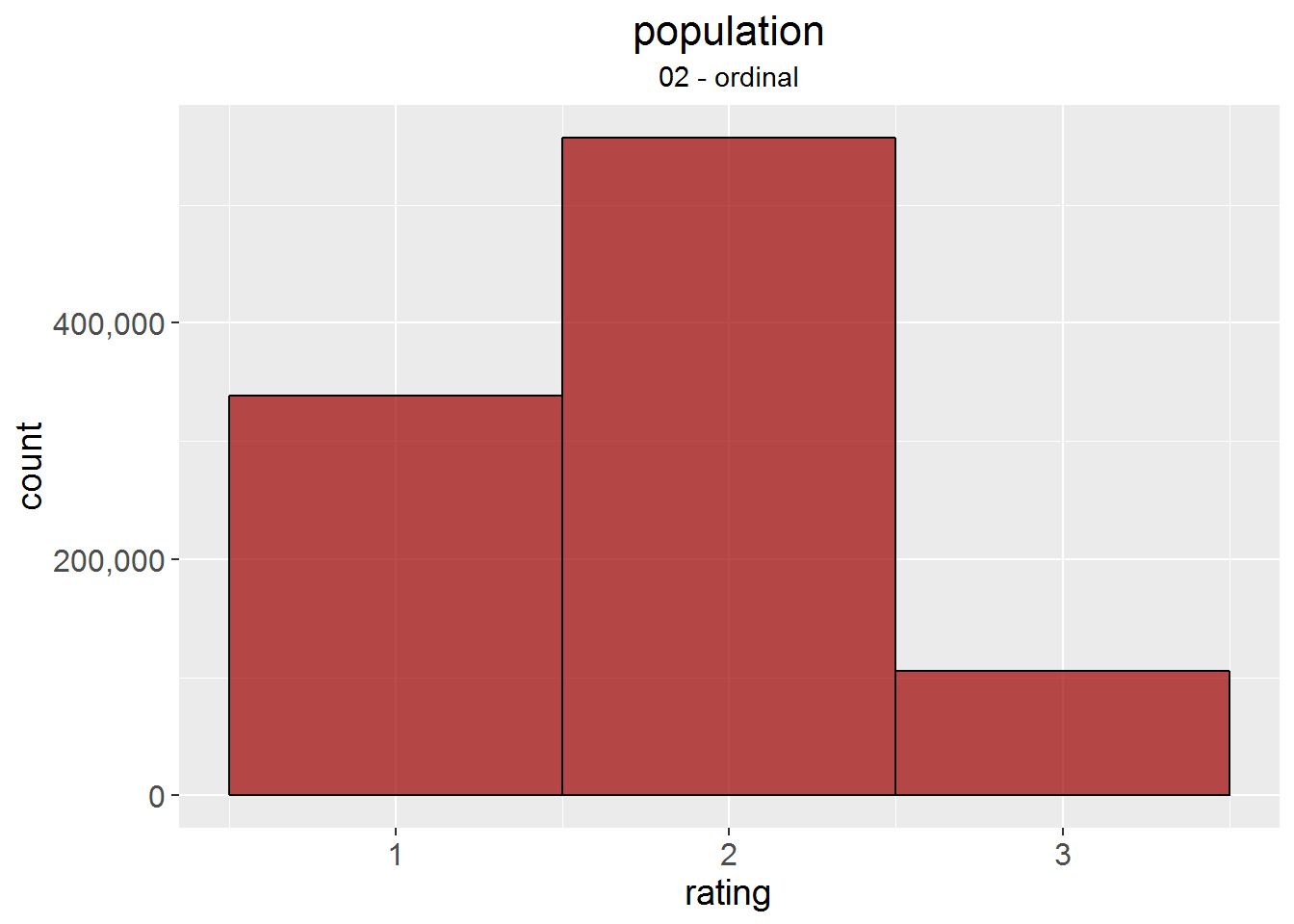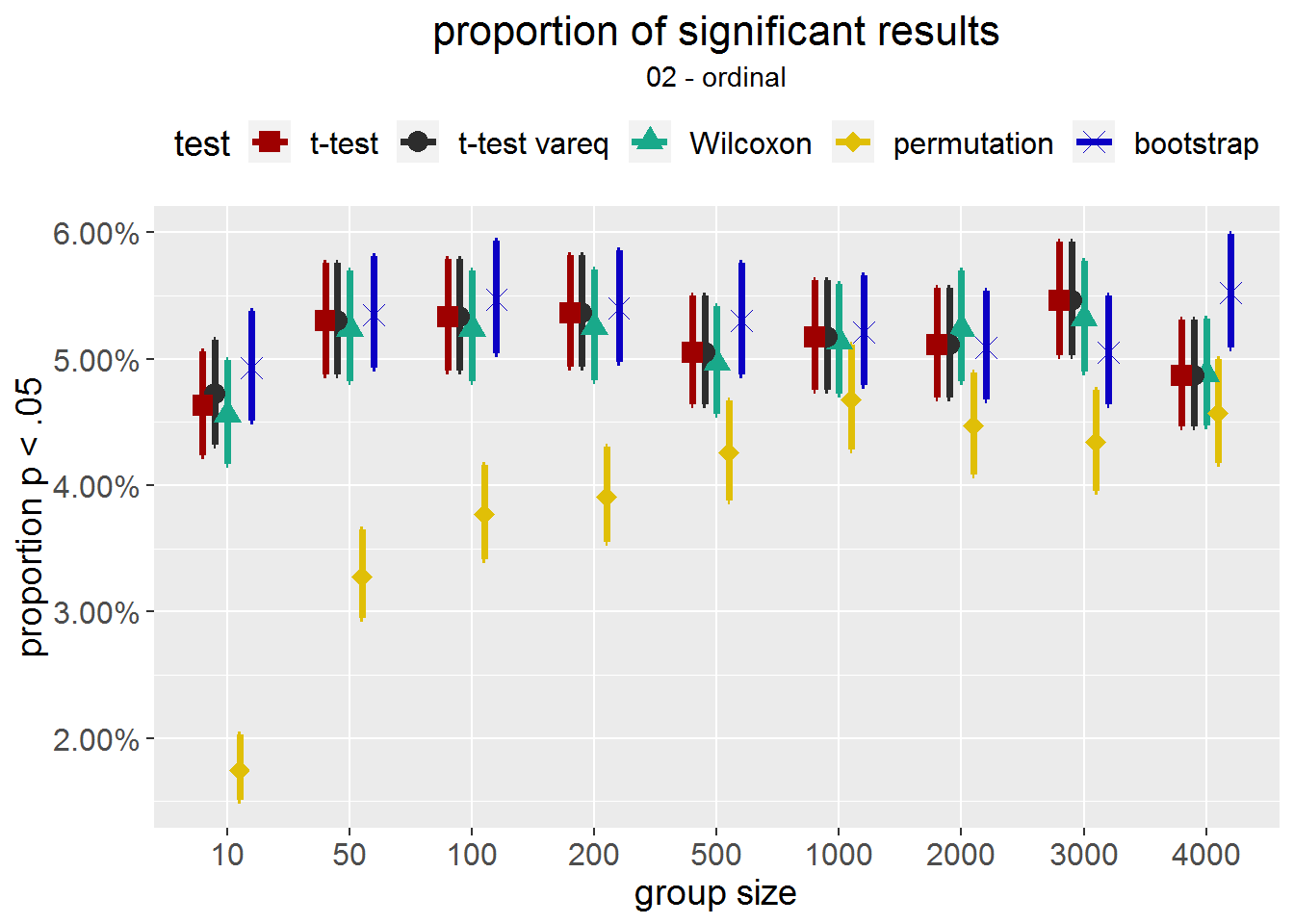## 03 - extremely skewed lognormal

Extremely skewed lognormal distribution (skewness = 7.48). Not converted to integers this time. Both populations: \(M = 1.65\), \(SD = 2.17\), median = 1.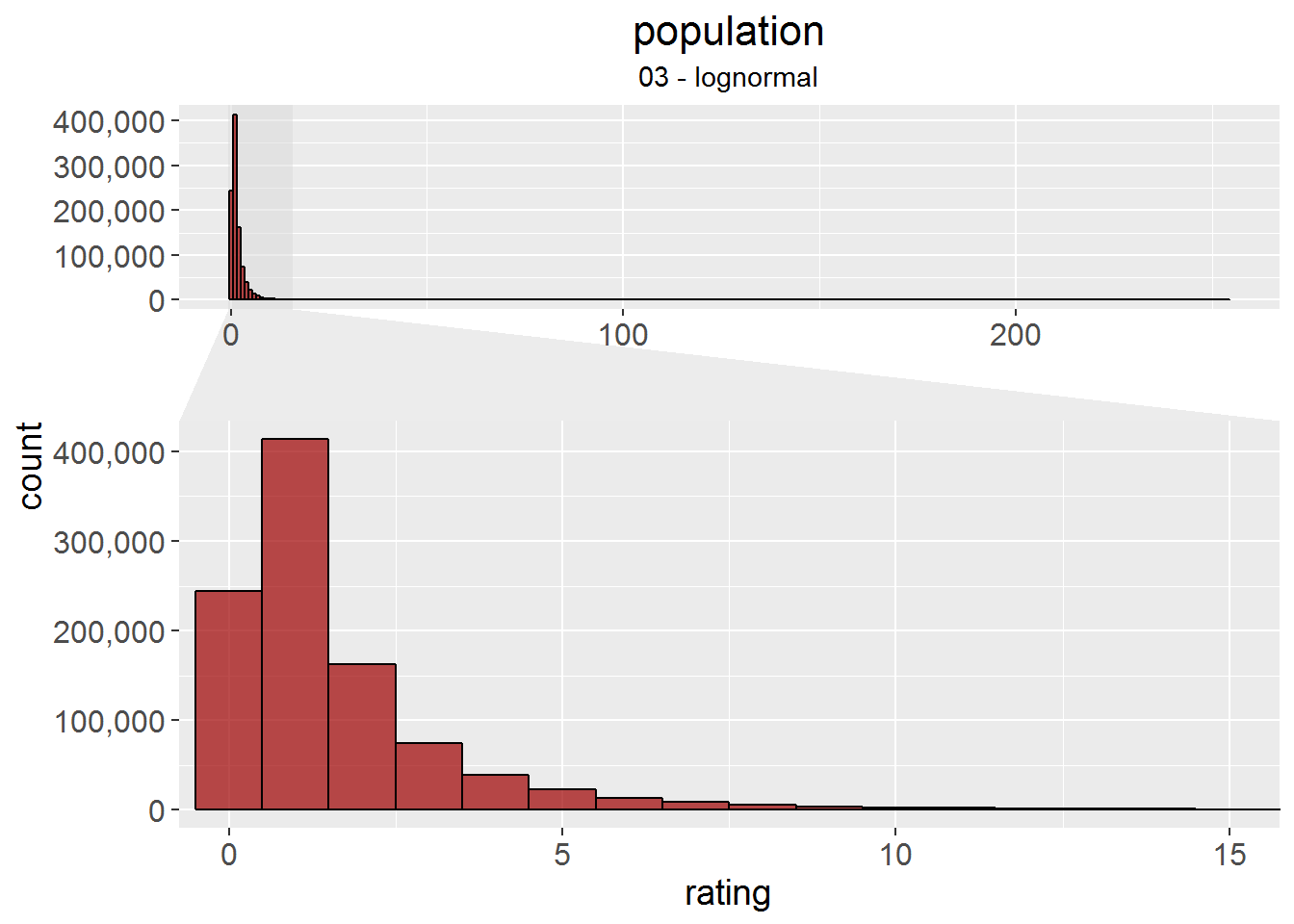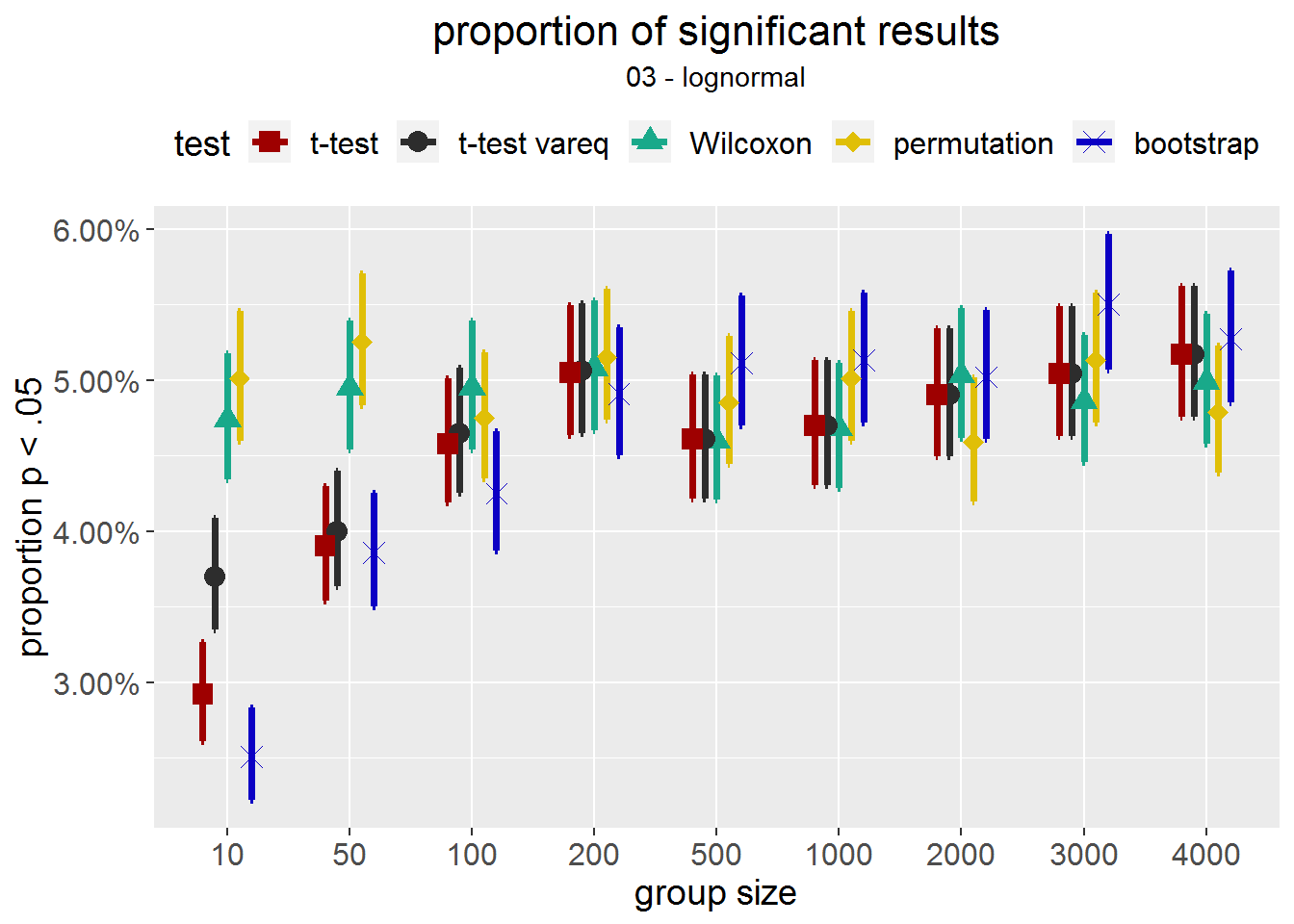## 04 - different SDs normal distribution

Different standard deviations between populations. Right after sampling: population 1 \(M = 5.50\), \(SD = 0.50\), median = 5.5, skewness = 0; population 2 \(M = 5.51\), \(SD = 3.00\), median = 5.5, skewness = 0. After conversion to integers from 1 - 10: population 1 \(M = 5.50\), \(SD = 0.58\), median = 6, skewness = 0; population 2 \(M = 5.50\), \(SD = 2.67\), median = 6, skewness = 0.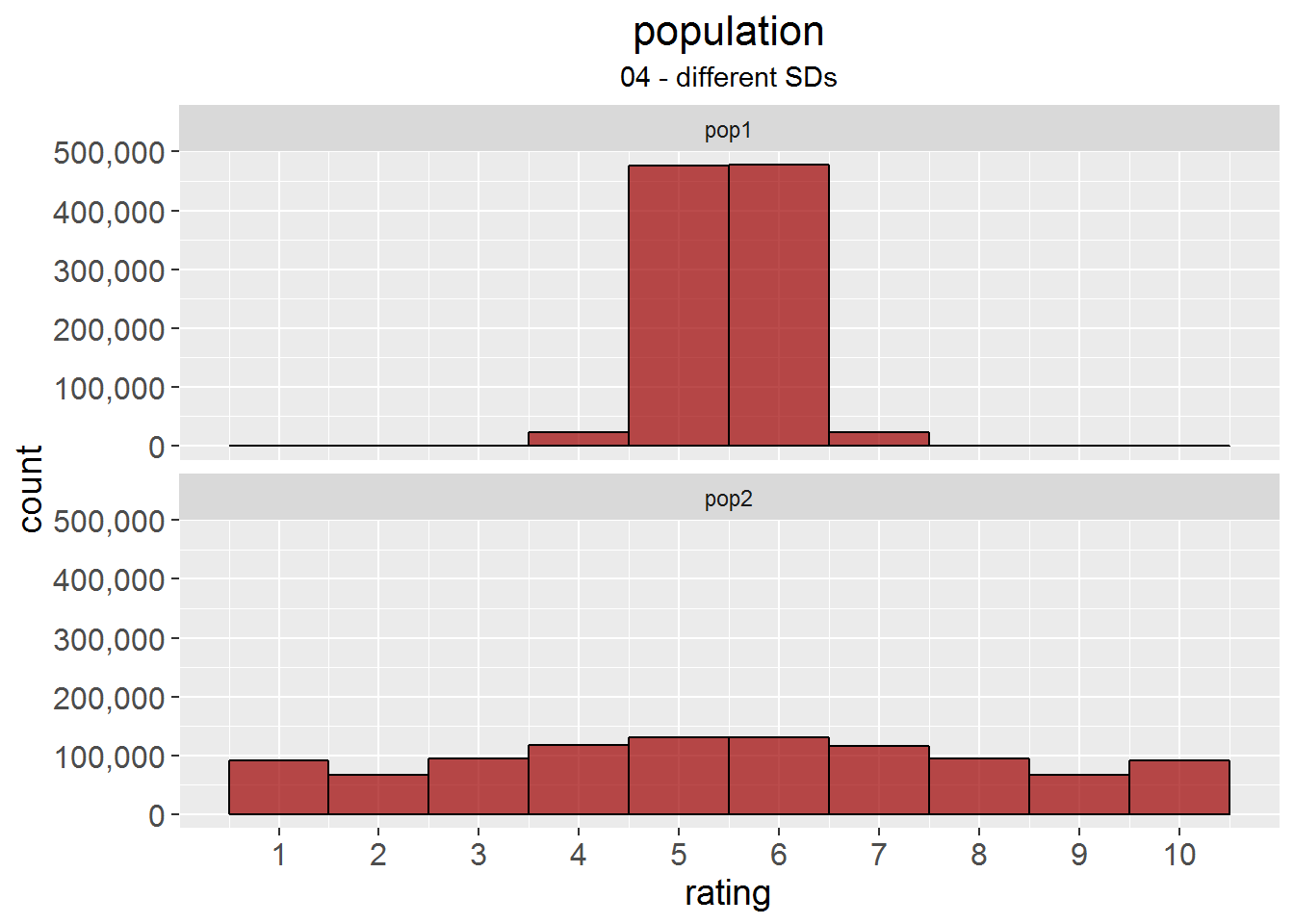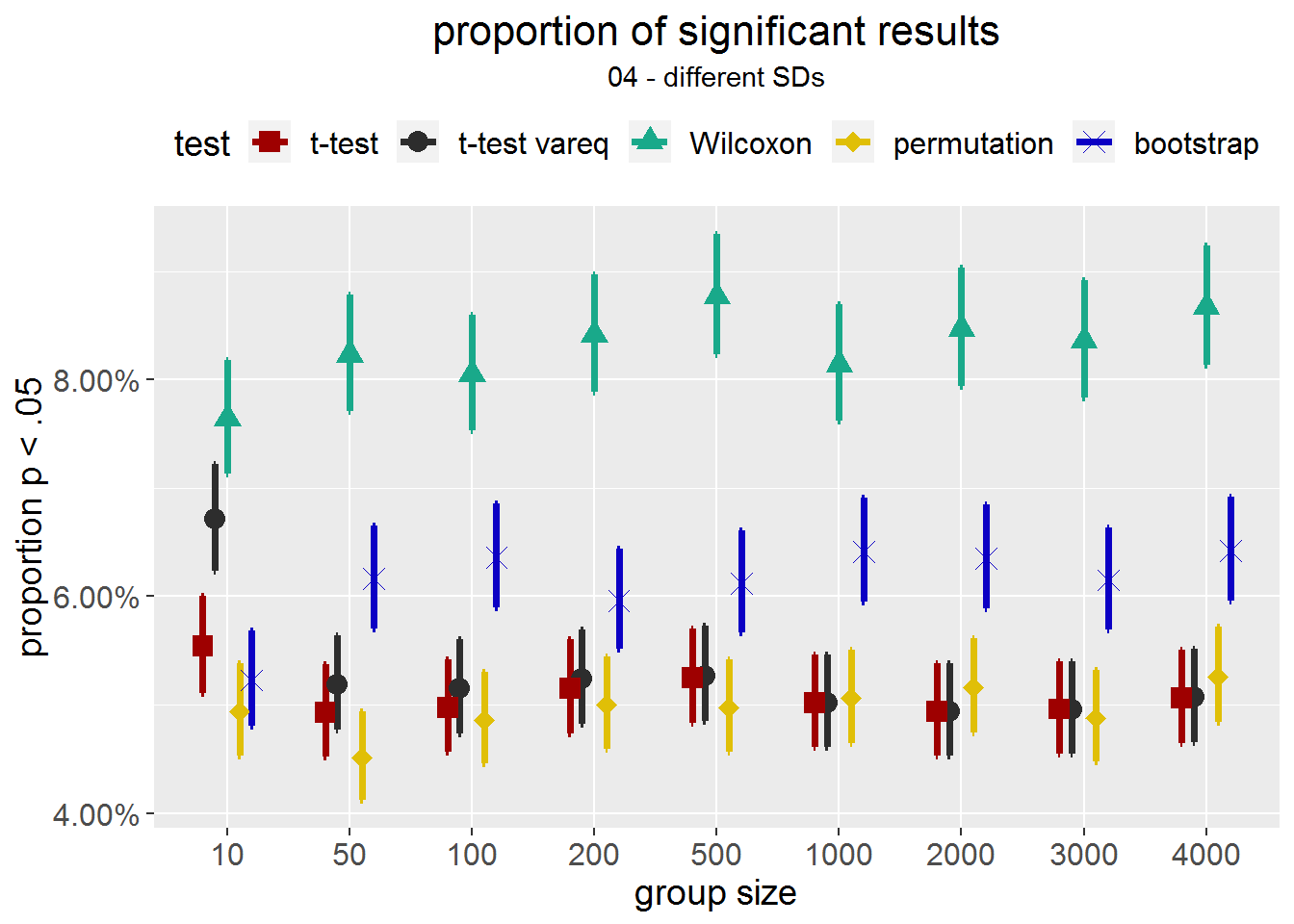## 04a - different SDs, still kinda normal

Initially, I tried to create lognormal distributions with a specified mean and sd (and the same median). To achieve the exakt same means and medians, I rounded the distribution. Turns out, they were rather normal than anything else. I included the example nevertheless: population 1 \(M = 40.00\), \(SD = 0.57\), median = 40, skewness = 0.02; population 2 \(M = 40.00\), \(SD = 1.04\), median = 40, skewness = 0.07.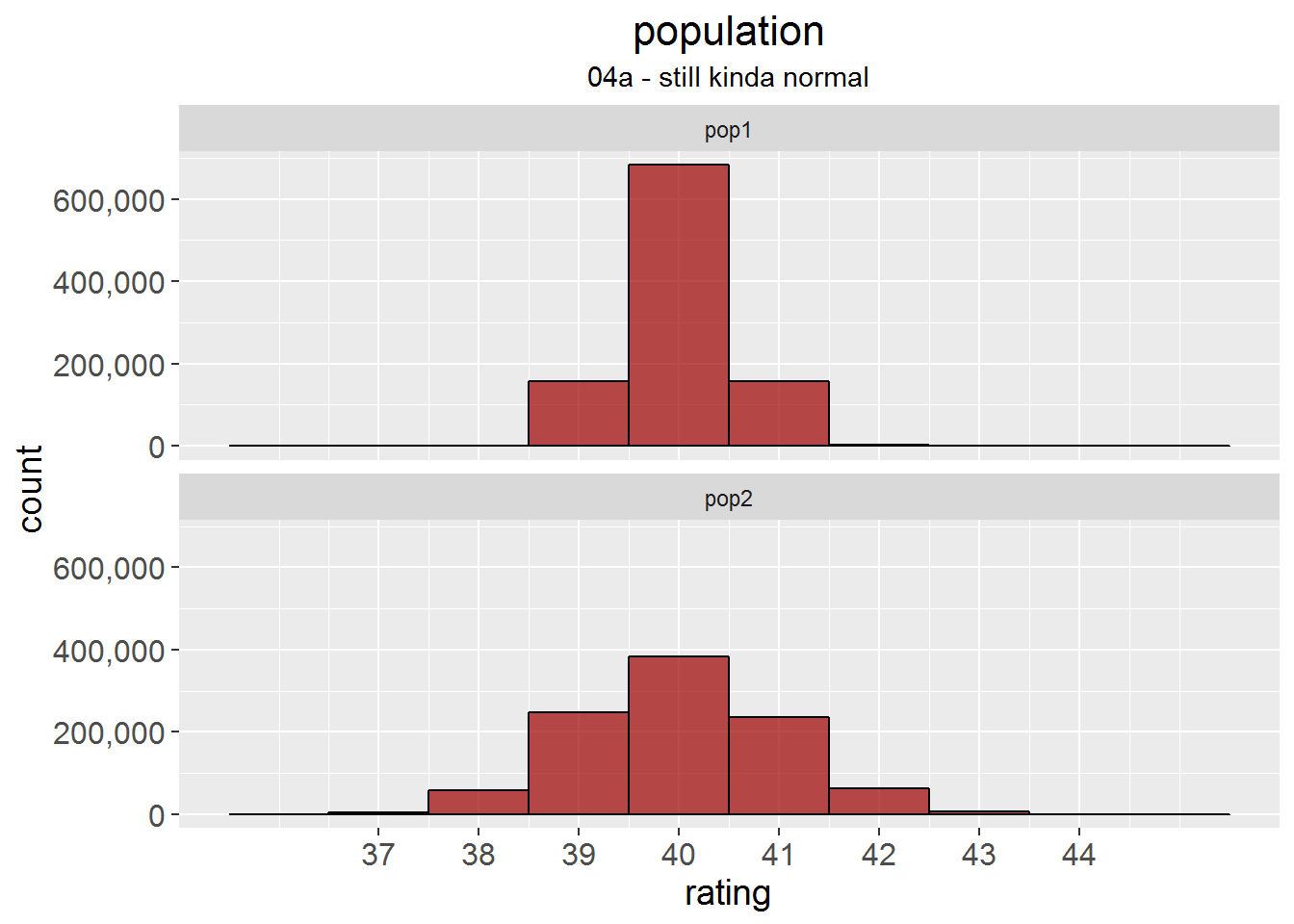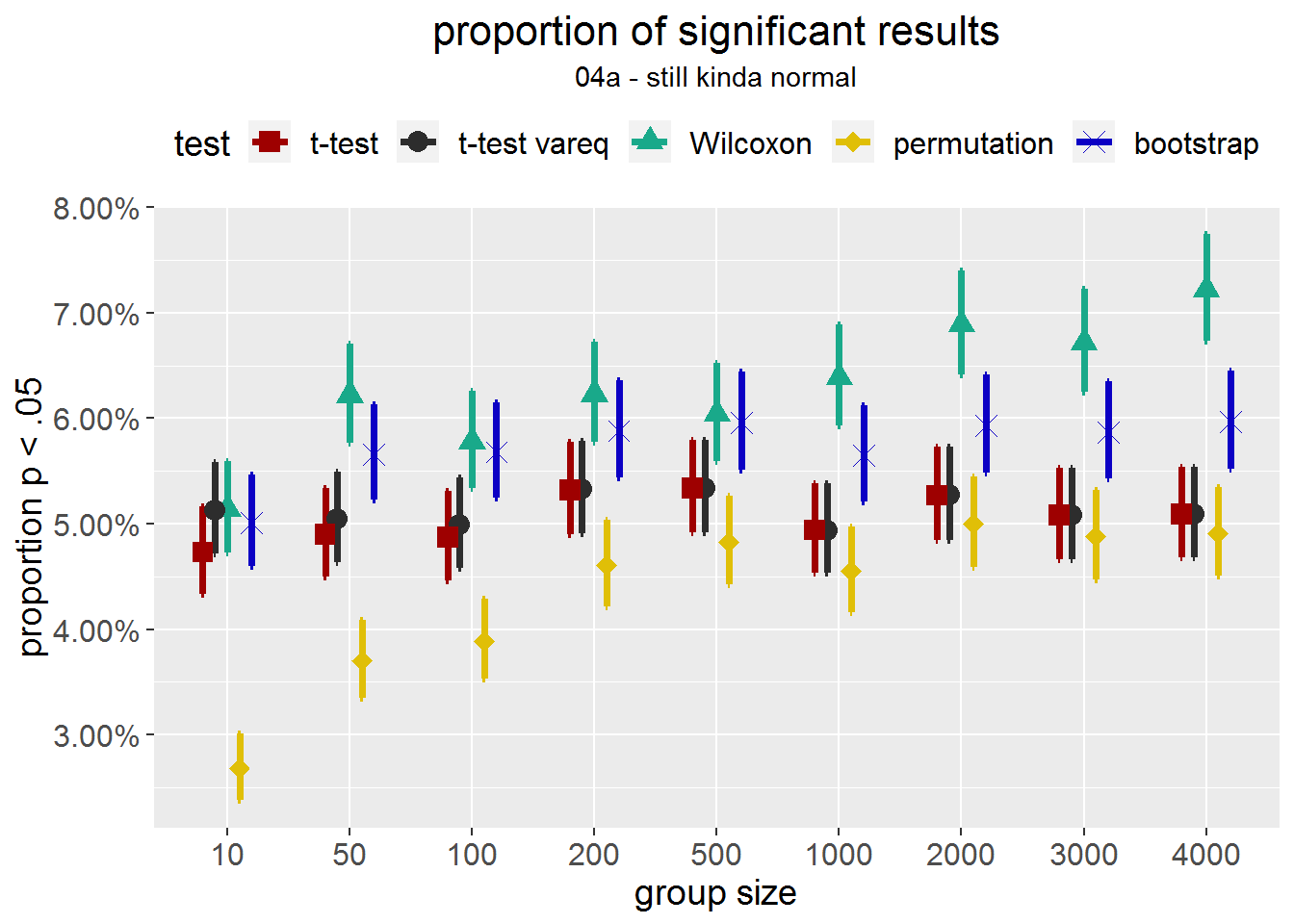## 05 - Different lognormal distributions

Similar to Fagerland: Two lognormal distributions with different SDs, but same mean and median: population 1 \(M = 40.00\), \(SD = 0.57\), median = 40, skewness = 0.02; population 2 \(M = 40.00\), \(SD = 1.04\), median = 40, skewness = 0.07.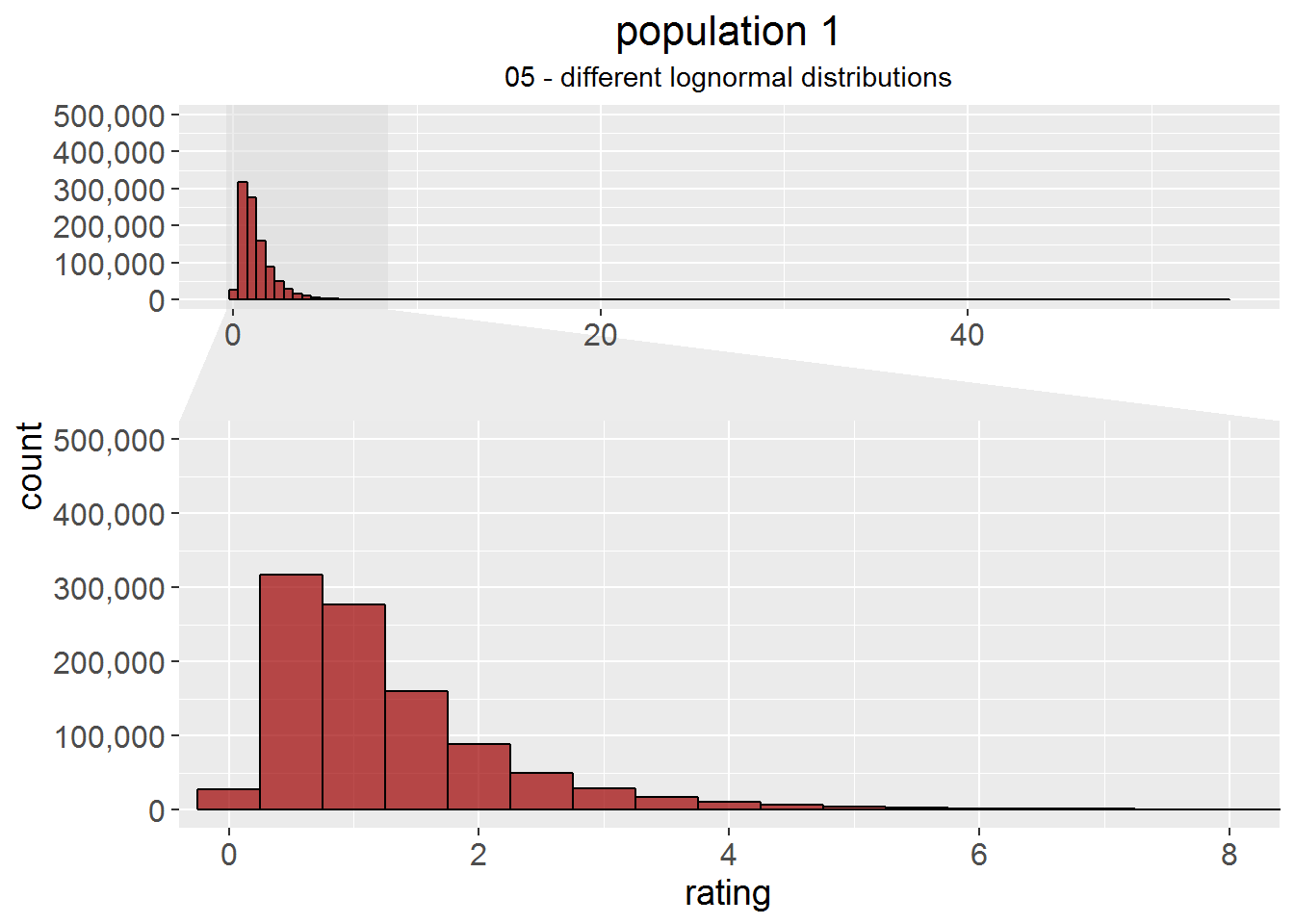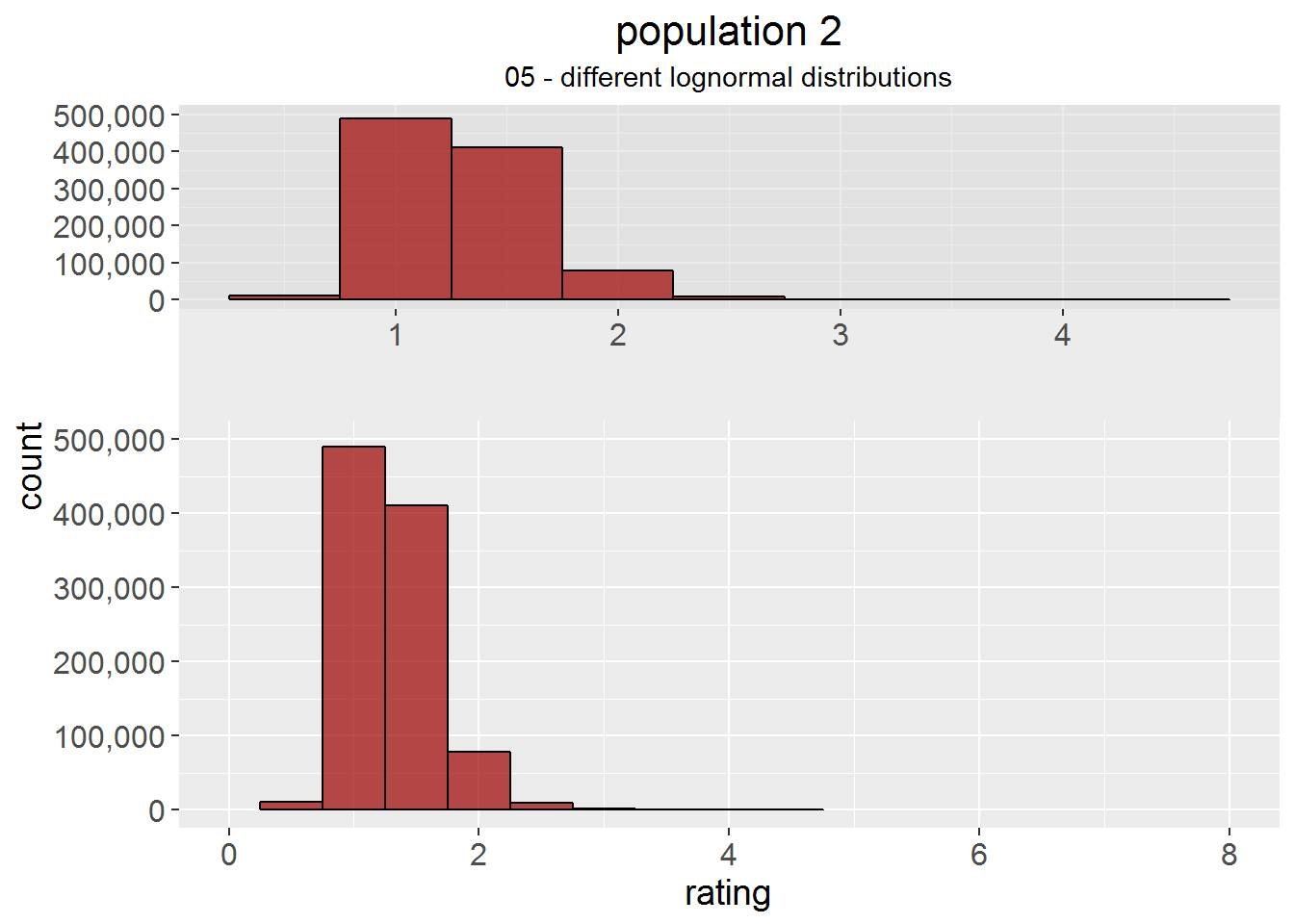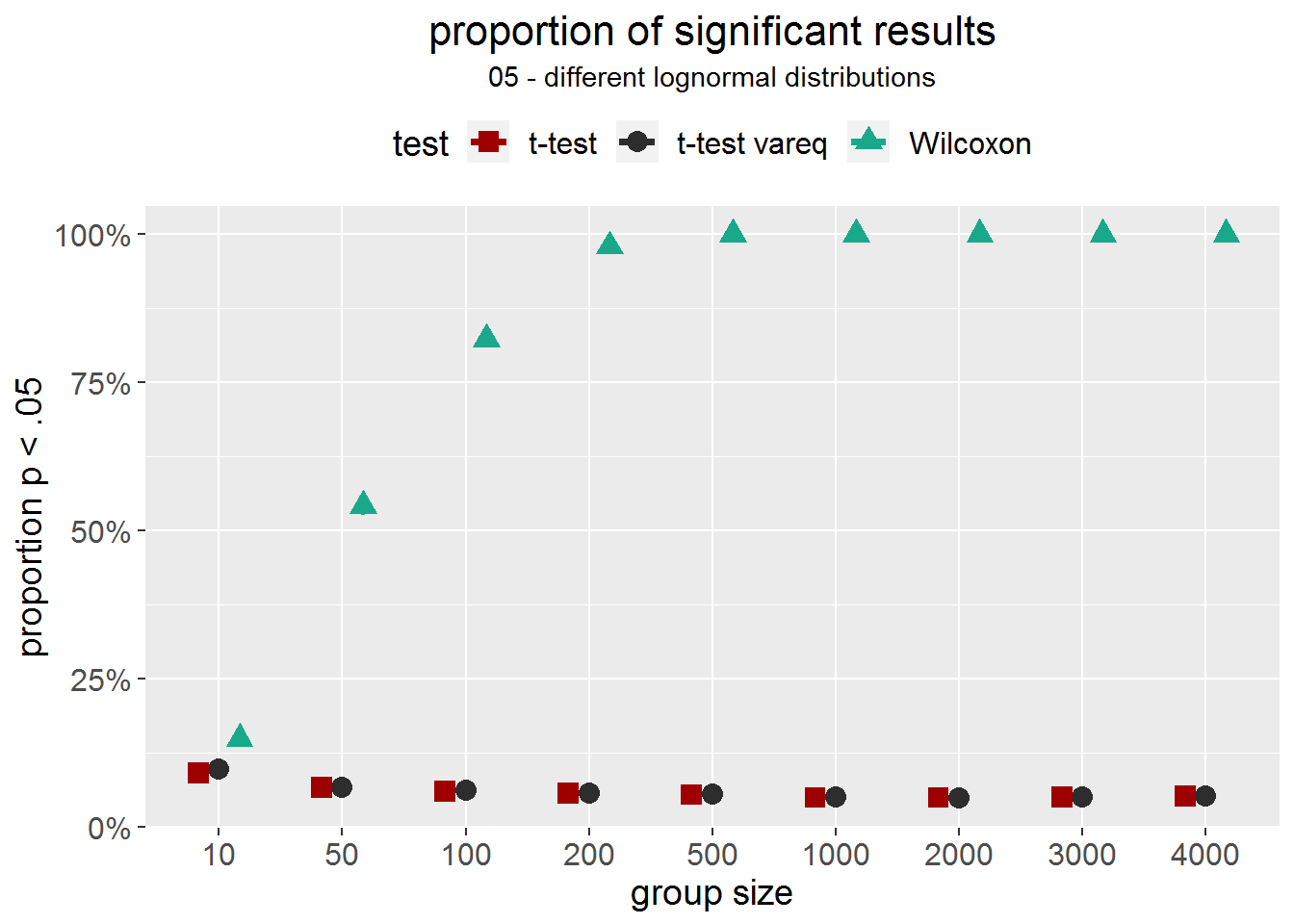## 06 - Two different gamma distributions

Similar to Fagerland: Two lognormal distributions with different SDs. Population 2 was shifted to the same mean as population 1. Note that Fagerland had a more elegant approach where the two distributions differed only in SD while mine also have a different skewness. population 1: \((M = 0.24\), \(SD = 0.49)\), skewness = 4.09; population 2: \((M = 0.24\), \(SD = 0.65)\), skewness = 3.07.